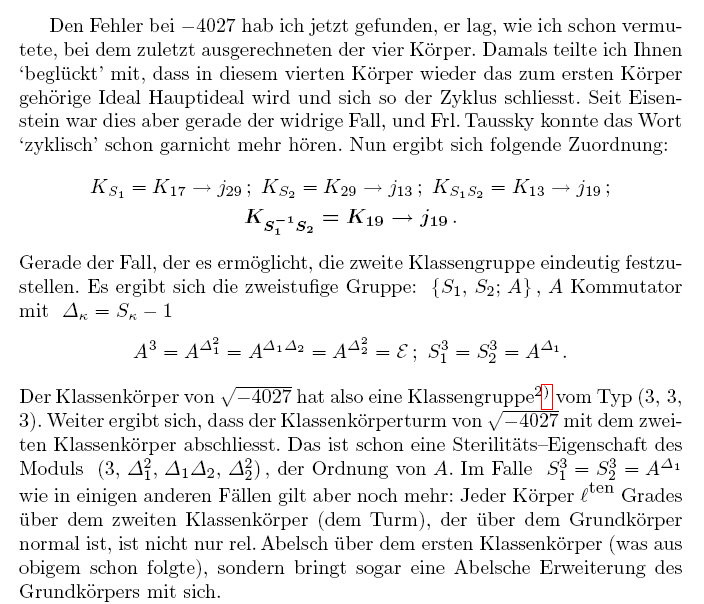# The complex quadratic base field K with discriminant d = -4027

This example has been investigated by Scholz and Taussky , Heider and Schmithals , Brink ,
and in our previous papers [4,5].

We give the complete data needed to determine the capitulation type and the group G=Gal(K2|K).
Computed in October, 1989, at the University of Graz, Computer Centre .

Counter, n = 2 Discriminant, d = -4027 3-class group of type (3,3) 3-class number, h = 9 Conductor, f = 1
The non-Galois absolute cubic subfields (L1,L2,L3,L4) of the four unramified cyclic cubic relative extensions N|K
Regulators, R 2.3 2.5 4.70 4.75
Class numbers, h 6 6 3 3
Polynomials, p(X) = X3 + C*X + D, with d(p) = i2*d
(C,D) (10,-1) (-44,-113) (43,-56) (-8,-15)
Indices, i 1 1 10 1
Fundamental units, e = (U + V*x + W*x2)/T, with P(x) = 0
U 0 -28 18 -7
V 1 -4 -13 2
W 0 1 -1 0
T 1 1 10 1
Splitting primes, q 43 13 19 61
Associated quadratic forms, F = a*X2 + b*X*Y + c*Y2
(a,b,c) (29,27,41) (13,9,79) (19,1,53) (17,11,61)
Represented primes, q 29, 43 13 19 17, 61
Associated ideal cubes, (x + y*d1/2)/2, with 4*q3 = x2 - d*y2
(x,y) (182,4) (69,1) (153,1) (125,1)
Principalization 2 3 3 1
Capitulation type D.10: (2,3,3,1) Group G in CBF1a(4,5) Contents

 References:  Arnold Scholz und Olga Taussky, Die Hauptideale der kubischen Klassenkörper imaginär quadratischer Zahlkörper, J. reine angew. Math. 171 (1934), 19 - 41  Franz-Peter Heider und Bodo Schmithals, Zur Kapitulation der Idealklassen in unverzweigten primzyklischen Erweiterungen, J. reine angew. Math. 336 (1982), 1 - 25  James R. Brink, The class field tower for imaginary quadratic number fields of type (3,3), Dissertation, Ohio State Univ., 1984.  Daniel C. Mayer, Dihedral fields of degree 2p, Univ. Graz, 1989.  Daniel C. Mayer, Principalization in complex S3-fields, Congressus Numerantium 80 (1991), 73 - 87  Daniel C. Mayer, Two-Stage Towers of 3-Class Fields over Quadratic Fields, (Latest Update) Univ. Graz, 2008.  Daniel C. Mayer, 3-Capitulation over Quadratic Fields with Discriminant |d| < 106 and 3-Class Group of Type (3,3), (Latest Update) Univ. Graz, Computer Centre, 2008.

Letters of Scholz to Hasse show the long and winding road
to the final results for this complex quadratic field:
(We gratefully acknowledge that these letters have been compiled and commented by Franz Lemmermeyer.)

October 24, 1927: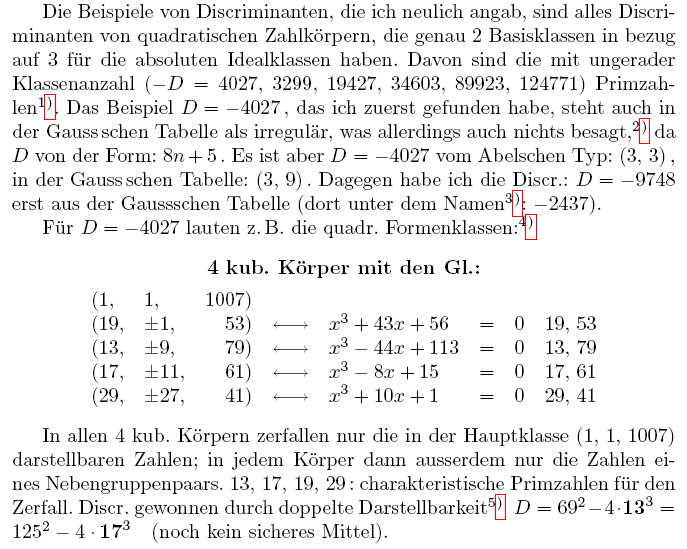October 15, 1928: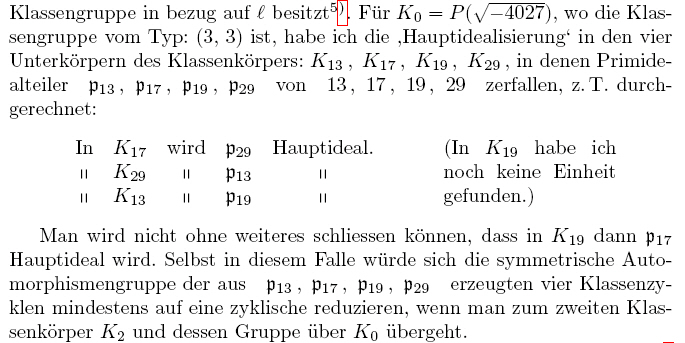October 24, 1928: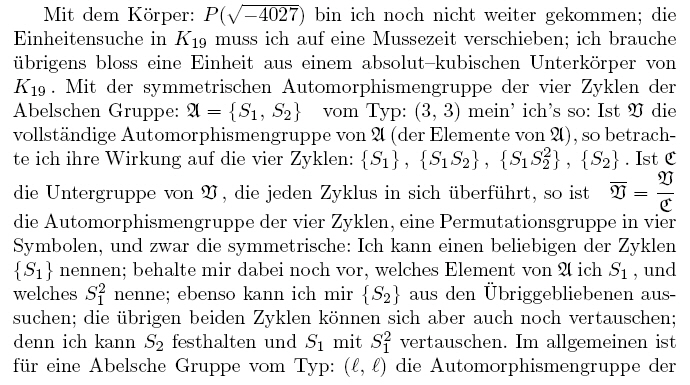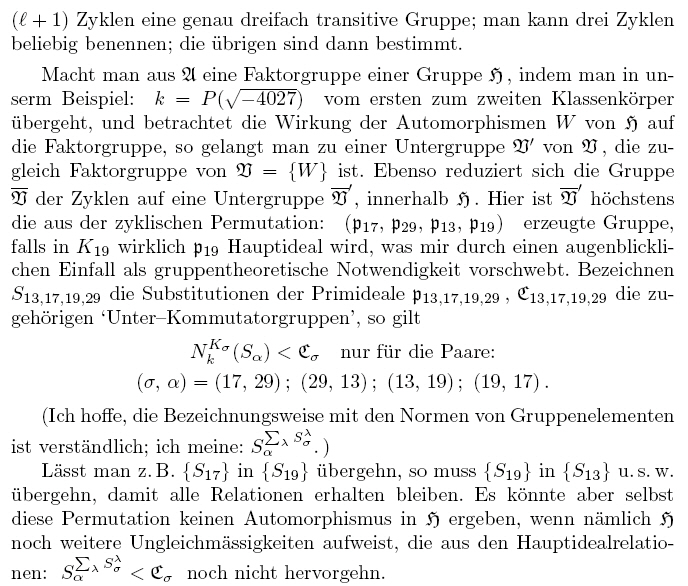October 12, 1930: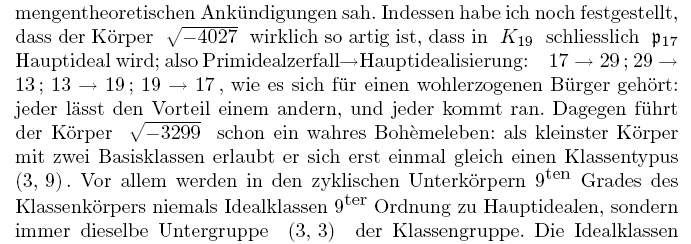November 29, 1931: